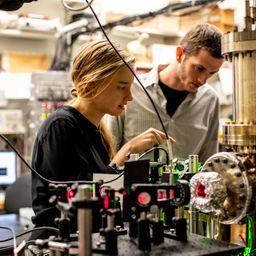## \begin{array}{l}{1.16 \times 10^{-3} \mathrm{m}} \\ {5.28 \times 10^{-32} \mathrm{m}}\end{array}

Quantum Physics

Atomic Physics

### Discussion

You must be signed in to discuss.
##### Top Physics 103 Educators##### Marshall S.

University of Washington##### Aspen F.

University of Sheffield

### Video Transcript

Okay, so on this problem, we're told that an electron and a bullet have a certain velocity and it's accurate with a certain person. And we want to get the visit. Uncertainty in the position of either object. Let's go ahead and write down our givens. So the velocity is just, I think, more simply written as 500 meters per second. Although I guess when scientific notation is clear that there's two sig figs. But maybe I'll put a dot here. And then there's 26 legs. And then we know that Delta Bi is gonna be, um, avoid creating a new symbol, Delta Bi. It's gonna be point a 1% over the 10.1% 0.1%. So, um 0.0, oh, oh! One times 500 in. So that's 1234 or so 500 times. 10 to the minus. For so 1234 So that's 0.5 meters per second here. All right. And the mass of the bullet is equal to plaint. Oh, too. Okay. Oh, point. Oh, tuo kilograms while I guess 36 legs. Oh, and then, of course, we have the mass of the electron. So then let's get a formula for Delta X. So we know that Delta acts times Delta P is equal to H over to pi. Delta P is equal to AM Belt Avi, and then we want to saturate the uncertainty by setting an equal because we want to find the minimum on certain team. And so then, if we want us now, solve her Delta acts, we get Delta X is equal to age over for pie and then times one over, um, Delta V. And now I'm going to go ahead and plug this into a calculator and then if we use, um, Delta X if we want to find it for the electron. Of course, we want to use the mass of the electron, but the velocity should stay the same. So let's go ahead and buy you a thought so that I get one point who won six times 10 to the minus three meters per second, not meters per second meters and with Delta X for the bullet Now we want to use pointed to. So it's going to significantly change your answer. 0.2 5.27 times seven minus 32 meters, so that's kind of astronomically small, so we know that, like, Okay, Yeah. We basically are gonna know the bullets position pretty well. According to quantum mechanics, you will check my sick bags that I stand by my sig figs. Great.University of Washington

#### Topics

Quantum Physics

Atomic Physics

##### Top Physics 103 Educators##### Marshall S.

University of Washington##### Aspen F.

University of Sheffield